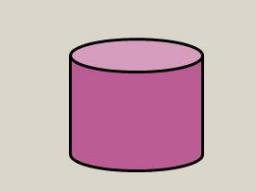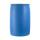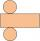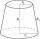# Cylinder diameter

The surface of the cylinder is 149 cm2. The cylinder height is 6 cm. What is the diameter of this cylinder?

Correct result:

D =  5.439 cm

#### Solution:

$S = 2\pi r^2 + 2\pi r h \ \\ S = \pi D^2/2 + \pi D h \ \\ \ \\ \pi D^2/2 + \pi D h - S = 0 \ \\ \pi D^2/2 + \pi \cdot 6 \cdot D - 149 = 0 \ \\ \ \\ 1.5707963267949D^2 +18.85D -149 =0 \ \\ \ \\ a=1.5707963267949; b=18.85; c=-149 \ \\ D = b^2 - 4ac = 18.85^2 - 4\cdot 1.5707963267949 \cdot (-149) = 1291.500369209 \ \\ D>0 \ \\ \ \\ D_{1,2} = \dfrac{ -b \pm \sqrt{ D } }{ 2a } = \dfrac{ -18.85 \pm \sqrt{ 1291.5 } }{ 3.1415926535898 } \ \\ D_{1,2} = -6 \pm 11.43924587037 \ \\ D_{1} = 5.4392458703697 \ \\ D_{2} = -17.43924587037 \ \\ \ \\ \text{ Factored form of the equation: } \ \\ 1.5707963267949 (D -5.4392458703697) (D +17.43924587037) = 0 \ \\ \ \\ D>0 \ \\ D \doteq 5.439 \ \text{cm}$We would be pleased if you find an error in the word problem, spelling mistakes, or inaccuracies and send it to us. Thank you!Tips to related online calculators

## Next similar math problems:

• Diameter = heightThe surface of the cylinder, the height of which is equal to the diameter of the base, is 4239 cm square. Calculate the cylinder volume.
• Volume and surfaceCalculate the volume and surface area of the cylinder when the cylinder height and base diameter is in a ratio of 3:4 and the area of the cylinder jacket is 24 dm2.
• Triangular prismThe triangular prism has a base in the shape of a right triangle, the legs of which is 9 cm and 40 cm long. The height of the prism is 20 cm. What is its volume cm3? And the surface cm2?
• Shell area cyThe cylinder has a shell content of 300 cm square, while the height of the cylinder is 12 cm. Calculate the volume of this cylinder.
• Tin with oilTin with oil has the shape of a rotating cylinder whose height is equal to the diameter of its base. Canned surface is 1884 cm2. Calculate how many liters of oil is in the tin.
• TankIn the middle of a cylindrical tank with a bottom diameter 251 cm is standing rod which is 13 cm above the water surface. If we bank rod its end reach surface of the water just by the tank wall. How deep is the tank?
• Conical bottleWhen a conical bottle rests on its flat base, the water in the bottle is 8 cm from it vertex. When the same conical bottle is turned upside down, the water level is 2 cm from its base. What is the height of the bottle?
• Height as diameter of baseThe rotary cylinder has a height equal to the base diameter and the surface of 471 cm2. Calculate the volume of a cylinder.
• Solid cuboidA solid cuboid has a volume of 40 cm3. The cuboid has a total surface area of 100 cm squared. One edge of the cuboid has length 2 cm. Find the length of a diagonal of the cuboid. Give your answer correct to 3 sig. Fig.
• Secret treasureScouts have a tent in the shape of a regular quadrilateral pyramid with a side of the base 4 m and a height of 3 m. Determine the radius r (and height h) of the container so that they can hide the largest possible treasure.
• The cylinderThe cylinder has a surface area of 300 square meters, while the height of the cylinder is 12 m. Calculate the volume of this cylinder.
• Cylindrical tank9.6 hl of water is poured into a cylindrical tank with a bottom diameter of 1.2 m. What height in centimeters does the water reach?
• The hollow cylinderThe hollow cylinder has a height of 70 cm, an outer diameter of 180 cm and an inner diameter of 120 cm. What is the surface of the body, including the area inside the cavity?
• Rotary cylinderThe rotating cylinder has a surface area 69.08 cm2. The area of the shell is 62.8 cm 2. What is the diameter of the cylinder?
• Hexagonal prism 2The regular hexagonal prism has a surface of 140 cm2 and height of 5 cm. Calculate its volume.
• Truncated cone 3The surface of the truncated rotating cone S = 7697 meters square, the substructure diameter is 56m and 42m, determine the height of the tang.
• Spherical cap 4What is the surface area of a spherical cap, the base diameter 20 m, height 2.5 m? Calculate using formula.